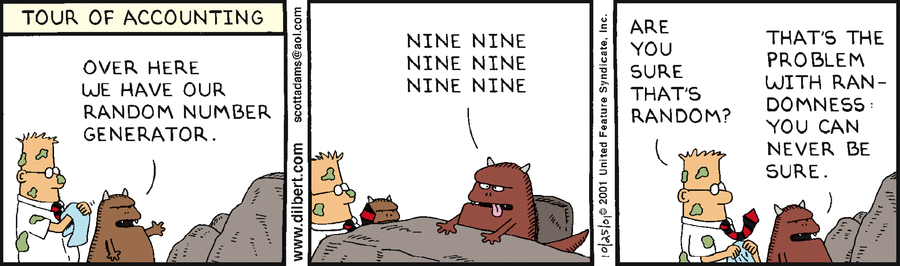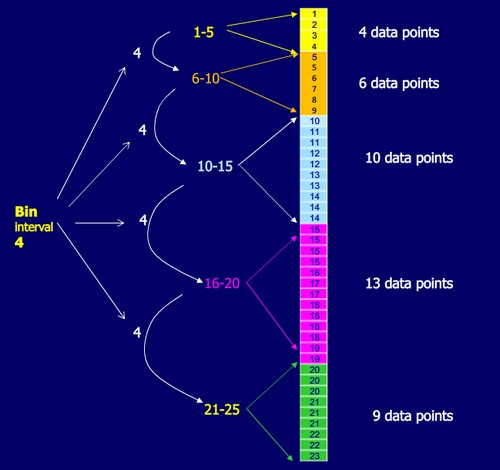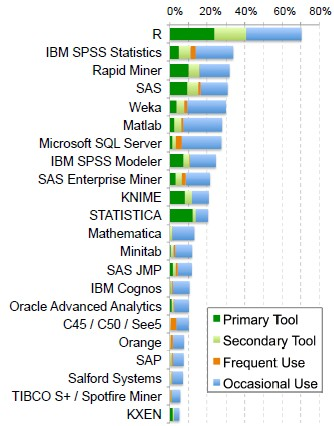# Data Mining - Entropy (Information Gain)

The degree to which a system has no pattern is known as entropy. A high-entropy source is completely chaotic, is unpredictable, and is called true randomness.

Entropy is a function “Information” that satisfies:where:

• p1p2 is the probability of event 1 and event 2
• p1 is the probability of an event 1
• p1 is the probability of an event 2where:

• H stands for entropy
• E for Ensemble ???

The entropy of a distribution with finite domain is maximized when all points have equal probability.

Bigger is the entropy, more is the event unpredicatble

Higher entropy means there is more unpredictability in the events being measured .

Higher entropy mean that the events being measured are less predictable.

100% predictability = 0 entropy


Fifty Fifty is an entropy of one.

Why is picking the attribute with the most information gain beneficial? It reduces entropy, which increases predictability. Information gain is positive when there is a decrease in entropy from choosing classifier/representation. A decrease in entropy signifies an decrease in unpredictability, which also means an increase in predictability.

## Example

### Flipping a Coin

A two class problem.### Rolling a die

A six class problem.

$$\begin{array}{rrl} Entropy & = & - \sum_{x}{ p_x log_2( p_x)} \\ & = & - 6 * (\frac{1}{6} log_2 (\frac{1}{6})) \\ & \approx & 2.58 \end{array}$$

### Rolling a weighted dieThe weighted die is more predictable than a fair die.

## How unpredictable is your data?

### Titanic

Titanic training set with a two class problem: survived or die

#### 342 survivors

case: 342 survivors on a total of 891 passengers#### 50 survivors

case: 50 survivors on a total of 891 passengersIt's a more predictable data set.

## Document / Reference

• Bill Howe (UW)

Recommended PagesCryptography - Key

A key is a parameter used in a cipher algorithm that determines the encryption operation (forward) and the decryption operation (backward). It's the only secret parameter that protect the anonymity...Data Mining - (Prediction|Guess)

Something predictable is showing a pattern and is therefore not truly random. entropytrue randomness Many forms of data mining model are predictive. For example, a model might predict income based on...Data Mining - Information Gain

Information theory was find by Claude_ShannonClaude Shannon. It has quantified entropy. This is key measure of information which is usually expressed by the average number of bits needed to store or communicate...Data Mining - Maximum Entropy Algorithm

Maximum Entropy (MaxEnt) models are feature-based classifier models. In a two-class scenario, it is the same as using logistic regression to find a distribution over the classes. MaxEnt makes no...Number - Random (Stochastic|Independent) or (Balanced)

Think of randomness as a lack of pattern. Something random should be unpredictable. We shouldn’t be able to predict the next value of the sequence The degree to which a system has no pattern is known...Statistics - (Discretizing|binning) (bin)

Discretization is the process of transforming numeric variables into nominal variables called bin. The created variables are nominal but are ordered (which is a concept that you will not find in true...Statistics - log-likelihood function (cross-entropy)

The “log-likelihood function” is a probabilistic function. The “log-likelihood function” is also referred to as the cross-entropyWeka

is an open-source project in machine learning, Data Mining. is a comprehensive collection of machine-learning algorithms for data mining tasks written in Java....